# 交易者生活窍门: 利用 defines (#define) 融合 ForEach

12 四月 2018, 07:26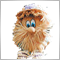0
11 009

— 兄弟, 什么能令你变酷？
— 兄弟, defines 预处理能令你变酷

(с)  fxsaber

### 一名好的程序员是一位懒惰的程序员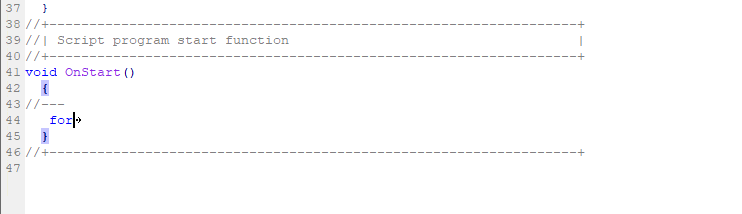```   int total=SymbolsTotal(true);
for(int i=0;i<total;i++)
{
string symbol=SymbolName(i,true);
PrintFormat("%d. %s",i+1,symbol);
}
```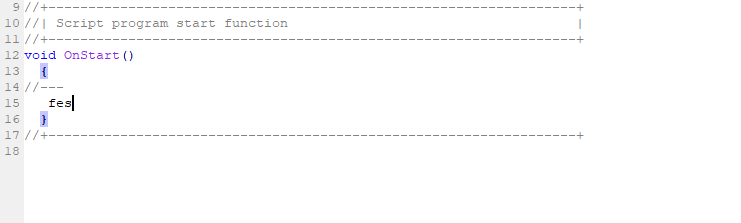MetaEditor 中没有自定义代码片段, 因此我们将利用 "defines"。 #define 宏定义替代是由追求多个目标的懒惰又聪明的程序员发明的。 其便利性包括便于阅读和编写复用代码。

```for(ulong order_id in History)
{
参照 order_id 工作
}
```

, 程序员的生活会更轻松一些。 您可以在互联网上找到这种方法的发对者和支持者。 在此我会告诉您如何用 #define 宏定义做类似的事情。

```#define ForEachSymbol(s,i)  string s; int total=SymbolsTotal(true); for(int i=0;i<total;i++,s=SymbolName(i,true))
//+------------------------------------------------------------------+
//| 脚本程序 start 函数                                               |
//+------------------------------------------------------------------+
void OnStart()
{
//---
ForEachSymbol(symbol,index)
{
PrintFormat("%d. %s",index,symbol);
}
}
```

```1. (null)
2. GBPUSD
3. USDCHF
4. USDJPY
...

```

'for' 语句由三个表达式和一条可执行语句组成:

 for(expression1; expression2; expression3)    statement;

Expression1 表示循环初始化。 Expression2 — 检查循环完成情况。 如果它为 'true', 则 for 循环实体操作符会被执行。 重复一切, 直到 expression2 变成 'false'。 如果它为 'false', 则循环终止并略过之下的语句。 ExpressionЗ 则在 每次迭代之后 进行计算。

```#define ForEachSymbol(s,i)  string s=SymbolName(0,true); int total=SymbolsTotal(true); for(int i=1;i<total;i++,s=SymbolName(i,true))
//+------------------------------------------------------------------+
//| 脚本程序 start 函数                                               |
//+------------------------------------------------------------------+
void OnStart()
{
//---
ForEachSymbol(symbol,index)
{
PrintFormat("%d. %s",index,symbol); // index+1 由 index 替代
}
}
```

```//+------------------------------------------------------------------+
//| 脚本程序 start 函数                                               |
//+------------------------------------------------------------------+
void OnStart()
{
//---
ForEachSymbol(symbol,index)
{
//--- 准备数据
double point=SymbolInfoDouble(symbol,SYMBOL_POINT);
long digits=SymbolInfoInteger(symbol,SYMBOL_DIGITS);
string str_point=DoubleToString(point,digits);
//--- 显示数据
digits," digits",", point=",str_point,")");
}
/* 简单输出
1. EURUSD spread=3 points (5 digits, point=0.00001)
2. USDCHF spread=8 points (5 digits, point=0.00001)
3. USDJPY spread=5 points (3 digits, point=0.001)
5. AUDUSD spread=5 points (5 digits, point=0.00001)
6. NZDUSD spread=10 points (5 digits, point=0.00001)
7. USDSEK spread=150 points (5 digits, point=0.00001)
*/
}
```

```#define ForEachObject(name,i)   string name=ObjectName(0,0); int total=ObjectsTotal(0); for(int i=1;i<=total;i++,name=ObjectName(0,i-1))
//+------------------------------------------------------------------+
//| 脚本程序 start 函数                                               |
//+------------------------------------------------------------------+
void OnStart()
{
//---
ForEachObject(objectname,index)
{
Print(index,": objectname=\"",objectname,"\", objecttype=",
EnumToString((ENUM_OBJECT)ObjectGetInteger(0,objectname,OBJPROP_TYPE)));
}
/* 简单输出
1: objectname="H1 Arrow 61067", objecttype=OBJ_ARROW_UP
2: objectname="H1 Rectangle 31152", objecttype=OBJ_RECTANGLE
3: objectname="H1 StdDev Channel 56931", objecttype=OBJ_STDDEVCHANNEL
4: objectname="H1 Trendline 6605", objecttype=OBJ_TREND
*/
}
```

ForEachObject 宏定义字符串变得有点长了。 此外, 理解单一字符串替换的代码更加困难。 但事实证明, 这个问题已经得到解决: 现在可以使用反斜杠 '\' 将宏定义切分为多个字符串。 结果如下:

```#define ForEachObject(name,i)   string name=ObjectName(0,0);   \
int ob_total=ObjectsTotal(0);                               \
for(int i=1;i<=ob_total;i++,name=ObjectName(0,i-1))
```

```#define ForEachOrder(ticket,i)    HistorySelect(0,TimeCurrent());    \
ulong ticket=OrderGetTicket(0);                                    \
int or_total=OrdersTotal();                                        \
for(int i=1;i<or_total;i++,ticket=OrderGetTicket(i))
//+------------------------------------------------------------------+
//| 脚本程序 start 函数                                               |
//+------------------------------------------------------------------+
void OnStart()
{
//---
ForEachOrder(orderticket,index)
{
Print(index,": #",orderticket," ",OrderGetString(ORDER_SYMBOL)," ",
EnumToString((ENUM_ORDER_TYPE)OrderGetInteger(ORDER_TYPE)));
}
/* 简单输出
2: #14246567 AUDNZD ORDER_TYPE_SELL_LIMIT
*/
}
```

```#define ForEachPosition(ticket,i) HistorySelect(0,TimeCurrent());    \
ulong ticket=PositionGetTicket(0);                                \
int po_total=PositionsTotal();                                    \
for(int i=1;i<=po_total;i++,ticket=PositionGetTicket(i-1))
//+------------------------------------------------------------------+
//| 脚本程序 start 函数                                               |
//+------------------------------------------------------------------+
void OnStart()
{
//---
ForEachPosition(positionid,index)
{
Print(index,": ",PositionGetString(POSITION_SYMBOL)," postionID #",positionid);
}
/* 简单输出
2: EURNZD postionID #13443909
3: AUDUSD postionID #14956799
4: EURUSD postionID #14878673
*/
```

```#define ForEachDeal(ticket,i) HistorySelect(0,TimeCurrent());        \
ulong ticket=HistoryDealGetTicket(0);                             \
int total=HistoryDealsTotal();                                    \
for(int i=1;i<=total;i++,ticket=HistoryDealGetTicket(i-1))
//+------------------------------------------------------------------+
//| 脚本程序 start 函数                                               |
//+------------------------------------------------------------------+
void OnStart()
{
//---
ForEachDeal(dealticket,index)
{
Print(index,": deal #",dealticket,",  order ticket=",
HistoryDealGetInteger(dealticket,DEAL_ORDER));
}
}
```

```#define ForEachHistoryOrder(ticket,i) HistorySelect(0,TimeCurrent());\
ulong ticket=HistoryOrderGetTicket(0);                            \
int total=HistoryOrdersTotal();                                   \
for(int i=1;i<=total;i++,ticket=HistoryOrderGetTicket(i-1))
//+------------------------------------------------------------------+
//| 脚本程序 start 函数                                               |
//+------------------------------------------------------------------+
void OnStart()
{
//---
ForEachHistoryOrder(historyorderticket,index)
{
Print(index,": #",historyorderticket);
}
}
```

```//+------------------------------------------------------------------+
//|                                                      ForEach.mqh |
//|                                版权所有 2018, MetaQuotes 软件公司 |
//|                                             https://www.mql5.com |
//+------------------------------------------------------------------+
#property copyright "版权所有 2018, MetaQuotes 软件公司"
//+------------------------------------------------------------------+
//| 市场观察搜索循环                                                  |
//+------------------------------------------------------------------+
#define ForEachSymbol(symbol,i)  string symbol=SymbolName(0,true);   \
int os_total=SymbolsTotal(true);                                  \
for(int i=1;i<os_total;i++,symbol=SymbolName(i,true))
//+------------------------------------------------------------------+
//| 搜索图表主窗口对象循环                                            |
//+------------------------------------------------------------------+
#define ForEachObject(name,i)   string name=ObjectName(0,0);         \
int ob_total=ObjectsTotal(0);                                     \
for(int i=1;i<=ob_total;i++,name=ObjectName(0,i-1))
//+------------------------------------------------------------------+
//| 搜索活跃订单循环                                                  |
//+------------------------------------------------------------------+
#define ForEachOrder(ticket,i)    HistorySelect(0,TimeCurrent());    \
ulong ticket=OrderGetTicket(0);                                   \
int or_total=OrdersTotal();                                       \
for(int i=1;i<or_total;i++,ticket=OrderGetTicket(i))
//+------------------------------------------------------------------+
//| 搜索持仓循环                                                      |
//+------------------------------------------------------------------+
#define ForEachPosition(ticket,i) HistorySelect(0,TimeCurrent());    \
ulong ticket=PositionGetTicket(0);                                \
int po_total=PositionsTotal();                                    \
for(int i=1;i<=po_total;i++,ticket=PositionGetTicket(i-1))
//+------------------------------------------------------------------+
//| 搜索历史交易循环                                                  |
//+------------------------------------------------------------------+
#define ForEachDeal(ticket,i) HistorySelect(0,TimeCurrent());        \
ulong ticket=HistoryDealGetTicket(0);                             \
int dh_total=HistoryDealsTotal();                                 \
for(int i=1;i<=dh_total;i++,ticket=HistoryDealGetTicket(i-1))
//+------------------------------------------------------------------+
//| 搜索历史订单循环                                       |
//+------------------------------------------------------------------+
#define ForEachHistoryOrder(ticket,i) HistorySelect(0,TimeCurrent());\
ulong ticket=HistoryOrderGetTicket(0);                            \
int oh_total=HistoryOrdersTotal();                                \
for(int i=1;i<=oh_total;i++,ticket=HistoryOrderGetTicket(i-1))
//+------------------------------------------------------------------+

```

```#define ForEachSymbolBack(symbol,i) int s_start=SymbolsTotal(true)-1;\
string symbol=SymbolName(s_start,true);                           \
for(int i=s_start;i>=0;i--,symbol=SymbolName(i,true))
//+------------------------------------------------------------------+
//| 脚本程序 start 函数                                               |
//+------------------------------------------------------------------+
void OnStart()
{
//---
ForEachSymbolBack(symbol,index)
{
//--- 准备数据
double point=SymbolInfoDouble(symbol,SYMBOL_POINT);
long digits=SymbolInfoInteger(symbol,SYMBOL_DIGITS);
string str_point=DoubleToString(point,digits);
//--- 输出数据
digits," digits",", point=",str_point,")");
}
/* 简单输出
3. USDJPY spread=5 points (3 digits, point=0.001)
2. USDCHF spread=8 points (5 digits, point=0.00001)
1. GBPUSD spread=9 points (5 digits, point=0.00001)
0. EURUSD spread=2 points (5 digits, point=0.00001)
*/
}
```

### 1. 本文中描述了哪些 MQL4 函数组

MQL4 AccountXXXX, MQL4 MarketInfo, MQL4 状态检查和 MQL4 预定义变量组将被转换为 MQL5。 所以, 四个文件: AccountInfo.mqhMarketInfo.mqhCheck.mqh 和 Predefined.mqh 要添加到 [数据文件夹]\MQL5\Include\SimpleCall\。 文件夹中有七个文件要从 MQL4 函数转换为 MQL5: AccountInfo.mqh, Check.mqh, IndicatorsMQL4.mqh, IndicatorsMQL5.mqh, MarketInfo.mqh, Predefined.mqhSeries.mqh

```//+------------------------------------------------------------------+
//|                                                  MACD Sample.mq4 |
//|                           版权所有 2005-2014, MetaQuotes 软件公司 |
//|                                              http://www.mql4.com |
//+------------------------------------------------------------------+
//---
#include <SimpleCall\SimpleCallMQL4.mqh>
//---
input double TakeProfit    =50;
```

1.1. MQL4 AccountXXXX

MQL4 AccountXXXX 函数已在文件中转换 [数据文件夹]\MQL5\Include\SimpleCall\AccountInfo.mqh

MQL4 MQL5 AccountInfoXXXX MQL5 CAccountInfo  注意
AccountInfoDouble  AccountInfoDouble  CAccountInfo::InfoDouble 或 CAccountInfo::double 方法
AccountInfoInteger  AccountInfoInteger  CAccountInfo::InfoInteger 或 CAccountInfo::integer 方法
AccountInfoString  AccountInfoString  CAccountInfo::InfoString 或 CAccountInfo::text 方法
AccountBalance  AccountInfoDouble(ACCOUNT_BALANCE) 或   CAccountInfo::Balance
AccountCredit  AccountInfoDouble(ACCOUNT_CREDIT) 或   CAccountInfo::Credit
AccountCompany  AccountInfoString(ACCOUNT_COMPANY) 或   CAccountInfo::Company
AccountCurrency  AccountInfoString(ACCOUNT_CURRENCY) 或   CAccountInfo::Currency
AccountEquity  AccountInfoDouble(ACCOUNT_EQUITY) 或   CAccountInfo::Equity
AccountFreeMargin  AccountInfoDouble(ACCOUNT_FREEMARGIN) 或   CAccountInfo::FreeMargin
AccountFreeMarginCheck  --- /---  CAccountInfo::FreeMarginCheck
AccountFreeMarginMode  无等价品  无等价品
AccountLeverage  AccountInfoInteger(ACCOUNT_LEVERAGE)   CAccountInfo::Leverage  在 MQL4 中, 它是 int 类型, 而在 MQL5 中, 它是 long
AccountMargin  AccountInfoDouble(ACCOUNT_MARGIN)  CAccountInfo::Margin
AccountName  AccountInfoString(ACCOUNT_NAME)  CAccountInfo::Name
AccountNumber  AccountInfoInteger(ACCOUNT_LOGIN)  CAccountInfo::Login  在 MQL4 中, 它是 int 类型, 而在 MQL5 中, 它是 long
AccountProfit  AccountInfoDouble(ACCOUNT_PROFIT)  CAccountInfo::Profit
AccountServer  AccountInfoString(ACCOUNT_SERVER)  CAccountInfo::Server
AccountStopoutLevel  AccountInfoDouble(ACCOUNT_MARGIN_SO_SO)  CAccountInfo::MarginStopOut  在 MQL4 中, 它是 int 类型, 而在 MQL5 中, 它是 double
AccountStopoutMode  AccountInfoInteger(ACCOUNT_MARGIN_SO_MODE)  CAccountInfo::StopoutMode

```//---
#define  OP_SELL                    ORDER_TYPE_SELL
//--- 返回当前帐户的余额值
```

#define 指令可用于将常用名称分配给常量。 有两种形式:

```#define identifier expression                   // 无参数形式
#define identifier(par1,... par8) expression    // 参数形式
```

#define 指令在源文本中将所有发现的 "identifier" 替换为 "expression"。

```//--- 返回当前帐户的余额值
#define  AccountBalance(void)       AccountInfoDouble(ACCOUNT_BALANCE)
//--- 返回当前帐户的信用值
#define  AccountCredit(void)        AccountInfoDouble(ACCOUNT_CREDIT)
//--- 返回当前帐户注册的经纪公司名称
#define  AccountCompany(void)       AccountInfoString(ACCOUNT_COMPANY)
//--- 返回当前帐户的货币名称
#define  AccountCurrency(void)      AccountInfoString(ACCOUNT_CURRENCY)
//--- 返回当前账户的净值
#define  AccountEquity(void)        AccountInfoDouble(ACCOUNT_EQUITY)
//--- 返回当前帐户的可用保证金
#define  AccountFreeMargin(void)    AccountInfoDouble(ACCOUNT_MARGIN_FREE)
```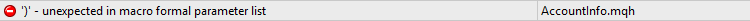```//--- 返回指定订单打开后剩余的可用保证金
//---    按当前账户的当前价格计算
double   AccountFreeMarginCheck(string symbol,int cmd,double volume)
{
double price=0.0;
price=SymbolInfoDouble(symbol,SYMBOL_BID);
//---
double margin_check=EMPTY_VALUE;
double margin=EMPTY_VALUE;
//---
return(AccountInfoDouble(ACCOUNT_FREEMARGIN)-margin_check);
}
```

```//--- 返回当前账户上开单的可用保证金计算模式
double AccountFreeMarginMode(void)
{
string text="MQL4 functions \"AccountFreeMarginMode()\" has no analogs in MQL5. Returned \"NAN - not a number\"";
Print(text);
return(double("nan"));
}
```

```//--- 返回当前账户的杠杆
#define  AccountLeverage(void)      (int)AccountInfoInteger(ACCOUNT_LEVERAGE)
//--- 返回当前帐户的保证金
#define  AccountMargin(void)        AccountInfoDouble(ACCOUNT_MARGIN)
//--- 返回当前帐户名称
#define  AccountName(void)          AccountInfoString(ACCOUNT_NAME)
//--- 返回当前帐号
//--- 返回当前帐户的利润值
#define  AccountProfit(void)        AccountInfoDouble(ACCOUNT_PROFIT)
//--- 返回连接的服务器名称
#define  AccountServer(void)        AccountInfoString(ACCOUNT_SERVER)
//--- 返回强制平仓级别的数值
#define  AccountStopoutLevel(void)  (int)AccountInfoDouble(ACCOUNT_MARGIN_SO_SO)
//--- 返回强制平仓级别的计算模式
int      AccountStopoutMode(void)
{
ENUM_ACCOUNT_STOPOUT_MODE stopout_mode=(ENUM_ACCOUNT_STOPOUT_MODE)AccountInfoInteger(ACCOUNT_MARGIN_SO_MODE);
if(stopout_mode==ACCOUNT_STOPOUT_MODE_PERCENT)
return(0);
return(1);
}
```

1.2. MQL4 MarketInfo

MQL4 MarketInfotXXXX 函数已在文件 [数据文件夹]\MQL5\Include\SimpleCall\MarketInfo.mqh 转换

MQL4 MarketInfo 是 double 类型, 但在 MQL5 中, MarketInfo 的等价品 SymbolInfoInteger() (得到 'long' 类型) 以及 SymbolInfoDouble() (得到 'double' 类型)。 此处, 我们可以清楚地感受到使用 MarketInfo (类型转换) 废弃函数的不便。 使用 MQL5 SYMBOL_DIGITS 作为示例:

MarketInfo(Symbol(),MODE_DIGITS) <= (double)SymbolInfoInteger(symbol,SYMBOL_DIGITS)

ID 描述

1.2.2. MQL4 MODE_SWAPTYPE 的歧义

MQL4 MODE_SWAPTYPE MQL5 ENUM_SYMBOL_SWAP_MODE
无等价品  SYMBOL_SWAP_MODE_DISABLED
0 - 按照点数  SYMBOL_SWAP_MODE_POINTS
1 - 按照品种的基准货币  SYMBOL_SWAP_MODE_CURRENCY_SYMBOL
3 - 按照保证金基准货币  SYMBOL_SWAP_MODE_CURRENCY_MARGIN
无等价品  SYMBOL_SWAP_MODE_CURRENCY_DEPOSIT
2 - 按照 %  SYMBOL_SWAP_MODE_INTEREST_CURRENT
2 - 按照 %  SYMBOL_SWAP_MODE_INTEREST_OPEN
无等价品  SYMBOL_SWAP_MODE_REOPEN_CURRENT
无等价品  SYMBOL_SWAP_MODE_REOPEN_BID

```/*
SYMBOL_SWAP_MODE_INTEREST_CURRENT

SYMBOL_SWAP_MODE_INTEREST_OPEN

*/
```

1.2.3. MQL4 MODE_PROFITCALCMODE 和 MODE_MARGINCALCMODE 的歧义

MQL5 的 ENUM_SYMBOL_CALC_MODE 枚举包括十个方法。 我们来比较 MQL4 的 MODE_PROFITCALCMODE 和 MQL5 的 ENUM_SYMBOL_CALC_MODE:

MQL4 MODE_PROFITCALCMODE "Forex" <==> MQL5 SYMBOL_CALC_MODE_FOREX

MQL4 MODE_PROFITCALCMODE "CFD" <==> MQL5 SYMBOL_CALC_MODE_CFD

MQL4 MODE_PROFITCALCMODE "Futures" <==> MQL5 SYMBOL_CALC_MODE_FUTURES

```...
...
case MODE_PROFITCALCMODE:
{
switch(profit_calc_mode)
{
case  SYMBOL_CALC_MODE_FOREX:
return((double)0);
case  SYMBOL_CALC_MODE_FUTURES:
return((double)2);
case  SYMBOL_CALC_MODE_CFD:
return((double)1);
default :
{
string text="MQL4 MODE_PROFITCALCMODE returned MQL5 "+EnumToString(profit_calc_mode);
Print(text);
return(double("nan"));
}
}
}
case MODE_MARGINCALCMODE:
{
switch(profit_calc_mode)
{
case  SYMBOL_CALC_MODE_FOREX:
return((double)0);
case  SYMBOL_CALC_MODE_FUTURES:
return((double)2);
case  SYMBOL_CALC_MODE_CFD:
return((double)1);
default :
{
string text="MQL4 MODE_MARGINCALCMODE returned MQL5 "+EnumToString(profit_calc_mode);
Print(text);
return(double("nan"));
}
}
}
```

1.3. MQL4 状态检查

• Digits
• Point
• IsConnected
• IsDemo
• IsDllsAllowed
• IsExpertEnabled
• IsLibrariesAllowed
• IsOptimization
• IsTesting
• IsVisualMode
• TerminalCompany
• TerminalName
• TerminalPath
MQL4 MQL5 MQL5 类 注意
Digits

MQL4 允许同时使用 Digits 和 Digits()
Point

MQL4 允许同时使用 Point 和 Point()
IsConnected  TerminalInfoInteger(TERMINAL_CONNECTED)  CTerminalInfo::IsConnected
IsDllsAllowed  TerminalInfoInteger(TERMINAL_DLLS_ALLOWED) and MQLInfoInteger(MQL_DLLS_ALLOWED)  CTerminalInfo::IsDLLsAllowed   TERMINAL_DLLS_ALLOWED 的状态最高, 而 MQL_DLLS_ALLOWED 可以忽略
IsLibrariesAllowed  MQLInfoInteger(MQL_DLLS_ALLOWED)  -/-  该检查毫无意义: 如果程序使用 DLL, 但您已禁用它们 (程序的依赖性选项卡), 那么您将无法运行该程序。
IsOptimization  MQLInfoInteger(MQL_OPTIMIZATION)  -/-  MQL5 程序有四种模式: 调试, 代码分析, 测试和优化
IsTesting  MQLInfoInteger(MQL_TESTER)  -/-  MQL5 程序有四种模式: 调试, 代码分析, 测试和优化
IsVisualMode  MQLInfoInteger(MQL_VISUAL_MODE)
MQL5 程序有四种模式: 调试, 代码分析, 测试和优化
TerminalCompany  TerminalInfoString(TERMINAL_COMPANY)  CTerminalInfo::Company
TerminalName  TerminalInfoString(TERMINAL_NAME)  CTerminalInfo::Name
TerminalPath  TerminalInfoString(TERMINAL_PATH)  CTerminalInfo::Path

```//+------------------------------------------------------------------+
//|                                                        Check.mqh |
//|                                版权所有 2018, MetaQuotes 软件公司 |
//|                                           http://wmua.ru/slesar/ |
//+------------------------------------------------------------------+
#property copyright "版权所有 2018, MetaQuotes 软件公司"
#property version   "1.003"
//--- 检查客户端和服务器之间的连接
#define IsConnected        (bool)TerminalInfoInteger(TERMINAL_CONNECTED)
//--- 检查智能交易系统是否在模拟账户上运行
//--- 检查是否允许智能交易系统的 DLL 函数调用
#define IsDllsAllowed      (bool)TerminalInfoInteger(TERMINAL_DLLS_ALLOWED)
//--- 检查是否允许智能交易系统运行
//--- 检查智能交易系统是否可以调用库函数
#define IsLibrariesAllowed (bool)MQLInfoInteger(MQL_DLLS_ALLOWED)
//--- 检查智能交易系统是否在策略测试器优化模式下运行
#define IsOptimization     (bool)MQLInfoInteger(MQL_OPTIMIZATION)
//--- 检查智能交易系统是否在测试模式下运行
#define IsTesting                (bool)MQLInfoInteger(MQL_TESTER)
//--- 检查智能交易系统是否允许交易, 且交易前后关联是否繁忙
//--- 返回有关交易前后关联的信息
//--- 检查智能交易系统是否在可视模式下进行测试
#define IsVisualMode          (bool)MQLInfoInteger(MQL_VISUAL_MODE)
//--- 返回拥有客户终端的公司名称
#define TerminalCompany    TerminalInfoString(TERMINAL_COMPANY)
//--- 返回客户端名称
#define TerminalName          TerminalInfoString(TERMINAL_NAME)
//--- 返回客户端启动的目录
#define TerminalPath          TerminalInfoString(TERMINAL_PATH)
//+------------------------------------------------------------------+
```

1.4. MQL4 预定义变量

MQL4 预定义变量:

• _Digits
• _Point
• _LastError
• _Period
• _RandomSeed
• _StopFlag
• _Symbol
• _UninitReason
• Bars
• Bid
• Close
• Digits
• High
• Low
• Open
• Point
• Time
• Volume

```//--- _Digits 变量存储小数点后的位数,
#define _Digits         Digits()
//--- _Point 变量包含报价货币中当前品种的点数大小
#define _Point          Point()
//--- _LastError 变量包含最后一个错误的代码
#define _LastError      GetLastError()
//--- _Period 变量包含当前图表的时间帧数值
#define _Period         Period()
//#define _RandomSeed
//--- _StopFlag 变量包含程序停止的标志
#define _StopFlag       IsStopped()
//--- _Symbol 变量包含当前图表的品种名称
#define _Symbol         Symbol()
//--- _UninitReason 变量包含程序未初始化原因的代码
#define _UninitReason   UninitializeReason()
//#define Bars            Bars(Symbol(),Period());
//#define Digits
//#define Point

```

MQL4 的 Ask 和 Bid 被替换为自定义 (自行编写) 的 GetAsk() 和 GetBid() 函数:

```//--- 当前品种最后知道的卖方价格 (ask 价格)
//--- 当前品种最后知道的买方价格 (报价, bid 价格)
#define Bid             GetBid()
...
{
MqlTick tick;
SymbolInfoTick(Symbol(),tick);
}
//--- 当前品种最后知道的买方价格 (报价, bid 价格)
double GetBid()
{
MqlTick tick;
SymbolInfoTick(Symbol(),tick);
return(tick.bid);
}
```

`#define Ask SymbolInfoDouble(__Symbol,SYMBOL_ASK)`

SymbolInfoDouble 的注释说:

'operator' 关键字将用于重载 (重新分配) [] 索引操作符。 这是将 MQL4 时间序列数组 (Open[], High[], Low[], Close[], Time[], Volume[]) 翻译为 MQL5 形式所必需的。

#define 指令可用于将常用名称分配给常量。 有两种形式:

```#define identifier expression                   // 无参数形式
#define identifier(par1,... par8) expression    // 参数形式
```

#define 指令在源文本中将所有发现的 "identifier" 替换为 "expression"。

```#define SeriesVolume(Volume,T) 159
//+-----------------------------------------------------------------+
//| 脚本程序 start 函数                                               |
//+------------------------------------------------------------------+
void OnStart()
{
long a=SeriesVolume(Volume,long);
}
```

`   long a=159;`

```//+------------------------------------------------------------------+
//|                                                      Test_en.mq5 |
//|                                           版权所有 2012, 公司名称 |
//|                                       http://www.companyname.net |
//+------------------------------------------------------------------+
#define SeriesVolume(Volume,T) class CVolume       \
{                                                \
public:                                          \
T operator[](const int i) const                \
{                                              \
long val;                                   \
if(CopyTickVolume(Symbol(),Period(),i,1,val)==1)\
return(val);                              \
else                                           \
return(-1);                                  \
}                                              \
};                                               \
CVolume Volume;
//---
SeriesVolume(Volume,long)
//+------------------------------------------------------------------+
//| 脚本程序 start 函数                                               |
//+------------------------------------------------------------------+
void OnStart()
{
Print(Volume);
}
//+------------------------------------------------------------------+

```

```//+------------------------------------------------------------------+
//|                                                      Test_en.mq5 |
//|                                           版权所有 2012, 公司名称 |
//|                                       http://www.companyname.net |
//+------------------------------------------------------------------+
class CVolume
{
public:
long operator[](const int i) const
{
long val;
if(CopyTickVolume(Symbol(),Period(),i,1,val)==1)
return(val);
else
return(-1);
}
};
CVolume Volume;
//+------------------------------------------------------------------+
//| 脚本程序 start 函数                                               |
//+------------------------------------------------------------------+
void OnStart()
{
Print(Volume);
}
```

iClose 的示例:

```//--- 返回指定品种、时间帧和偏移柱线的收盘价
double   iClose(
string                    symbol,              // 品种
ENUM_TIMEFRAMES           timeframe,           // 时间帧
int                       shift                // 偏移
)
{
double result=0.0;
//---
double val;
ResetLastError();
int copied=CopyClose(symbol,timeframe,shift,1,val);
if(copied>0)
result=val;
else
Print(__FUNCTION__,": CopyClose error=",GetLastError());
//---
return(result);
}
```

2. 其它文件中的修改

```double NaN=double("nan");
#define MODE_MAIN          0
#define MODE_SIGNAL        1
#define MODE_PLUSDI        1
#define MODE_MINUSDI       2
#define MODE_GATORJAW      1
#define MODE_GATORTEETH    2
#define MODE_GATORLIPS     3
#define MODE_UPPER         1
#define MODE_LOWER         2
#define MODE_TENKANSEN     1
#define MODE_KIJUNSEN      2
#define MODE_SENKOUSPANA   3
#define MODE_SENKOUSPANB   4
#define MODE_CHIKOUSPAN    5
```

```#define MODE_OPEN    0
//#define MODE_LOW     1
//#define MODE_HIGH    2
#define MODE_CLOSE   3
#define MODE_VOLUME  4
//#define MODE_TIME    5
```

```//+------------------------------------------------------------------+
//|                                版权所有 2018, MetaQuotes 软件公司 |
//|                                           http://wmua.ru/slesar/ |
//+------------------------------------------------------------------+
#property copyright "版权所有 2018, MetaQuotes 软件公司"
//---
#define MODE_LOW     10001
#define MODE_HIGH    10002
#define MODE_TIME    10005
```

### 结束语

• 访问指标时返回错误的处理限制;
• 在同时访问多于一个指标时降低测试速度;
• 根据连接的是 IndicatorsMQL5.mqh 或 IndicatorsMQL4.mqh, 需要正确高亮指示线;
• 无法调试 #define 宏替代;
• 没有参数化的 #define 参数工具提示;
• 隐藏在宏定义后面的变量会有潜在冲突。

• 代码编写简单 — 一个字符串替换多个字符串;
• 可视性和简洁性 — 代码量越少, 理解起来就越容易;
• 宏替换以红色突出显示, 因此可以更轻松地查看用户 ID 和函数;
• 能够开发自定义代码片段等价品。

ForEachSymbol.mq5 (3.73 KB)
ForEachObject.mq5 (2.82 KB)
ForEachOrder.mq5 (2.59 KB)
ForEachDeal.mq5 (1.24 KB)
SimpleCall.zip (24.17 KB)

#### 该作者的其他文章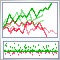在 MetaTrader 5 中交易策略优化的可视化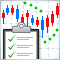如何创建订购指标的需求规范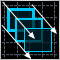如何创建任意复杂度的图形面板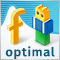利用文斯 (Vince) 进行资金管理。 作为 MQL5 向导模块实现